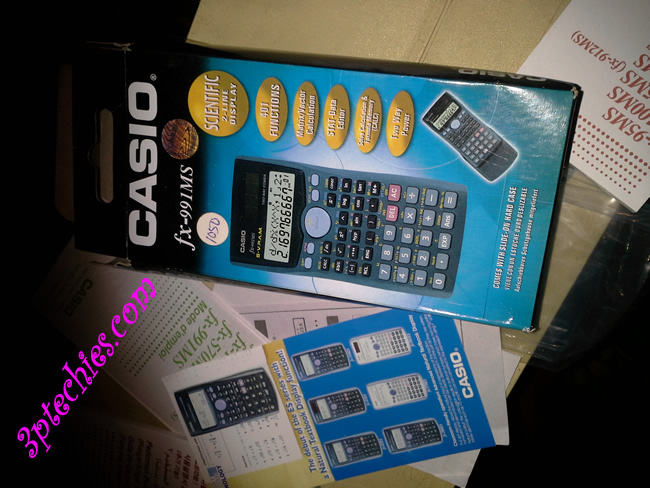# Casio fx-991MS Scientific Calculator Hands-on Review

0
##### Casio fx-991MS 2-Line display Scientific Calculator \$13
5

Summary

Casio fx-991MS 2-Line display Scientific Calculator is A 2-line display Scientific Calculator from Casio

Sending
##### User Review
3 (1 vote)

Casio FX-991MS 2-Line display scientific calculator is a very efficient scientific calculator with more than 400 functions. It is one of the very best models around today as it can be used for solving various mathematical and scientific equations.

A female colleague enjoined me help her with her mathematical problems which I normally do using a computer software that makes solving scientific & mathematical equations easy and effortless.

On knowing how limited the software could be for word problems and complicated equations, I decided to shop for a decent scientific (Engineering) calculator.## Unboxing and Features

After purchasing the calculator from a nearby bookshop, I unboxed it, went through the voluminous manual and operation guide to see what new features are added or removed.

After two months of usage, I concluded that this device is worth a review as it betters the other scientific calculators I have used in time past.I used Porpo before going for Casio and must say that the decision was actually a wise one. From basic mathematical equations, scientific calculations to matrix calculations, metric conversions and statistics, Casio fx-991MS Scientific Calculator offers all basic functions an engineer will need to attend to mathematical and scientific equations.

•   401 Functions
• 2-Line Display
• Matrix / Vector Calculation
• Stat-Data Editor (Standard Deviation, Normal Distribution Calculations, Regression Analysis)
• Multi-replay Function
• Solve Calculation & Formula Memory (CALC)
• Slide-on Hard Case
• Two Way Power (Solar plus Battery)
• S-V.P.A.M. (Super Visually Perfect Algebraic Method)
• 10 + 2 digits (10-digit mantissa + 2-digit exponential display.)
• Dot matrix display
• 9 variable memories
• Combination and permutation
• Fraction calculations

## Tech Spec

• Brand Name – Casio
• Model No – fx-991MS
• Type – Scientific
• Display (Digits) – 12
• Power Source – Battery and Solar
• Key Type – Plastic
• Battery Size – AA

### Price

Casio FX-991MS 2-Line display scientific calculator goes for about \$13 at Amazon and sells for about NGN1,200 in Nigerian bookshops and electronic dealers shop.

It is a steal for this amount considering the robust functions and advanced features of this model.

### Trick and Tweaks

There are some new trick and tweaks for use on the Casio FX-991MS 2-Line display scientific calculator.

These tricks are just the basic  built-in features of the calculator that are not entirely obvious, because the previous generation of scientific calculators did not have these features.

I stumbled on these tweaks when trying to explore the possibilities with the device and what best way to fully use the 401 functions it comes with.